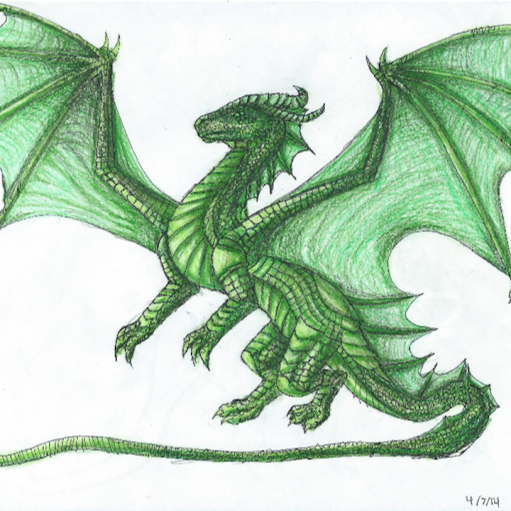12:14 AM
A new tag was created by Rodrigo de Azevedo, including a tag-excerpt.
0I heard that each element $a(i,j)$ of the matrix either represents the degree from vertex $i$ to vertex $j$ or does it represent the weight?

0Does there exist a multigraph $G$ of order $8$ such that the minimal $d(G) = 0$ while maximal $d(G) = 7$? What if ‘multigraph $G$’ is replaced by ‘graph $G$’? Answer: such multigraph does not exist, but graph?

4An edge set of a graph is a set of doubletons, pairing edges. For example: has an edge set of $\{\{6,4\},\{4,5\},\{4,3\},\{5,2\},\{5,1\},\{3,2\},\{1,2\}\}$. A set, by definition, cannot have duplicate elements, else it is not a set. is a multigraph. All graphs have edge sets, yet the edge s...

3Let we have a directed multigraph such that or every its vertex the set of edges from this vertex is finite and ordered (in other words, numbered $1,\dots,n$). I need this construct to describe (depth-first or breath-first) traversing this directed multigraph (where edges are iterated in the abo...

1The answer to this question could be trivial! The line graph of simple $d$-regular graph is ($2d-2$)-regular, since each edge is connected to $d-1$ edges for each of its two vertices. My question is the line graph of regular multigraph is also regular graph? I think that only the loops coul...

2According to Wikipedia: [...] a bridge in an undirected graph is an edge whose deletion increases the number of connected components. Equivalently, an edge is a bridge if and only if it is not contained in any cycle. Suppose I have a multigraph $G = (V,E)$ with two vertices $u,v \in V$ with...

0If I have a graph that has an edge that straight connects vertice $A$ to $B$ and another that connects vertice $A$ to $C$ then to $B$ is it considered a multigraph? Clarification will be much appreciated. Thanks.

1Is there a good algorithm for doing a topological sort of a subgraph of a multigraph? More specifically, given a multigraph G and a node n in the graph. Consider the subgraph G' all the nodes reachable from n in G. Is there a good way to find a topological sort of G'? For example A --> B -->...

2I'm an undergraduate student majoring in mathematics. I'm solving my home assignment and got stuck at this problem. Suppose $d_1\geq d_2\geq\dots \geq d_n\geq 1$ and $\sum_{i=1}^nd_i$ is even. Prove that there is a multigraph with degree sequence $d_1, d_2, > \dots, d_n$ if and only if $d_1... 1In directed multigraph, two vertices,$a$and$b$, may have zero or more parallel edges connecting them in one direction or the other. Does this set of parallel edges have a name? I seem to remember reading a book which gave this set a name. 2The net-degree of a vertex$v$, denoted$\text{netdeg}(v)$, in a digraph$G$is defined by $$\text{netdeg}(v)=| ~ \text{outdeg}(v) - \text{indeg}(v) ~|$$ where$\text{outdeg}(v)$and$\text{indeg}(v)$are the out-degree and in-degree of$v$. Show that the edges of any multigraph may be orien... 2suppose you have the$K_{2n}$covered by a multigraph consisting of$2n-1$bicliques, each consisting of a partition of the vertex set into two sets of equal size. Here is a picture of$K_{6}\$ with 5 bicliques. My question is: under which circumstances is it possible to pick a perfect matchi...